Back | Home

Introduction to
artificial neural networks (ANNs)

The world today

Maybe you have heard here and there about some technological wonders based on artificial neural networks -- about intelligent robots, smart weapons or even stock trading advisory systems? What's going on? Are we really living at the turning point of history where machines are taking over and mankind will be doomed? To be honest, it is not completely impossible, but artificial neural networks in their current state of evolution are hardly the right target for accusations of that kind. The most complex neural networks of our time are not working in the dark corners of high-tech labs. No, they are in our heads.

Start thinking!

How much do we know about the inner mechanisms of our brain? How often do we think about how exactly we think about the things we think about? The fact that this sentence looks a bit weird should be considered alarming as it shows the lack of discussions on the topic. Think about it!

Biology

Human brain is an extremely complex structure. Its workings can be discussed on a huge number of different levels -- starting from the low end of atoms and molecules and going up to the social interactions of global scale. All these levels are remarkably interesting, but as our current concern is artificial neural networks, we will go to the cellular level.

The main component of our biological neural net is a cell called neuron. Many different types of neurons are present in brain, but their general structure is mostly the same: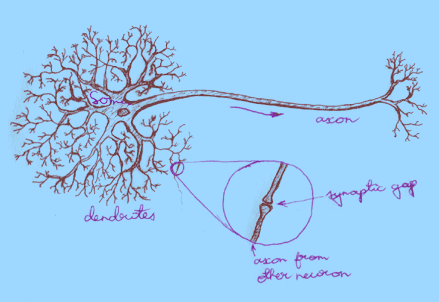On the drawing you can see the main parts of a neural cell:

• Dendrites collect incoming signals. They have a lot of branches and their surface is quite irregular. Dendrites got their name because of a tree-like structure.

•
• Soma is the body of the neural cell. It processes incoming signals (and, of course, performs all the activities necessary for a cell to survive).

•
• Axon transmits signals to the dendrites of other neurons. Axon has less branches, smoother surface and greater length than dendrites.

•
• Synapses are connections between neurons. They are actually tiny gaps that allow signals to jump from an axon to a dendrite.
The main principle of neuron's work is already visible from the description of its structure -- neuron collects signals, processes them and feeds into other neurons. In reality, however, everything is very complex. First of all, signals are far from simplicity. They are passed via electrochemical processes based on different ions (mainly sodium (Na), potassium (K) and chloride (Cl)). Even worse, the information is coded not into the amplitude of signals, but instead into frequency of these electrochemical pulsations (although that doesn't automatically mean we could just forget about the amplitude). Secondly, although the scales are microscopic, the numbers are macroscopic. Each neuron has up to 10,000 input connections via dendrites, all passing signals into the soma. And the number of such neurons in a brain is estimated to be somewhere between 10 and 100 billion (thats 10,000,000,000 and 100,000,000,000)! Finally, all this unimaginably huge system is in constant change --  new connections are being formed, some cells stop functioning due to aging, etc.

Technology

Todays technology is nowhere near the complexity described in previous paragraph, neither in numbers nor in principles. Although artificial neural networks DO have some resemblance to their biological counterparts, they are still tiny and simple structures. Their simplicity, however, does NOT mean they are fully understood and easily described by mathematical tools.

Artificial neuron

There are a great many different models of neurons and neural networks, lots of them being successfully used in various applications. Still, most of them have a lot in common and differ only in details. The most widespread structure of an artificial neuron is: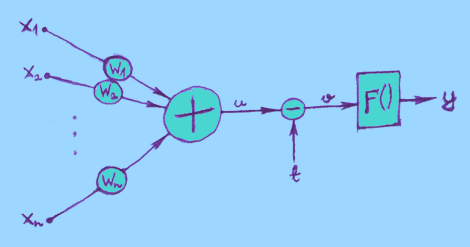If it looks a bit messy and scary first, then don't worry, actually it is very simple.

• On the left there are inputs x1, x2, ..., xn.
•
• Each input goes through a connection, wich has a certain weight w, and is multiplied by that weight: x * w.
•
• All these multiplied values are then added together:
• u = x1*w1 + x2*w2 + ... + xn*wn

• This sum u is then adjusted with threshold t:
• v = u - t

• And finally a so called "activation function" F() is applied to the adjusted sum, giving us the output of neuron:
• y = F(v)

In general all these parameters and variables (inputs, weights, threshold) can be both positive or negative (and zero, too). Negative threshold is often called with a different name: bias.

There are three basic types of activation function F():

1. Threshold function (not to confuse with threshold parameter t):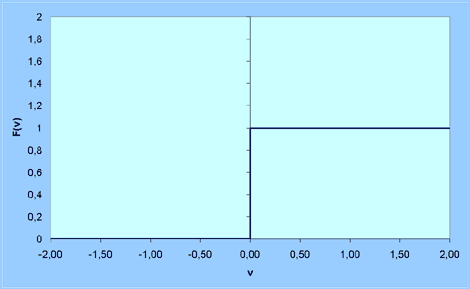F(v) = 0,  if v < 0
F(v) = 1,  if v = 0 or v > 0

2. Piecewise-Linear function: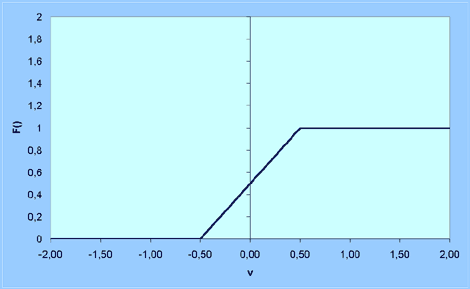F(v) = 0,  if v < -0.5 or v = -0.5
F(v) = v + 0.5,  if -0.5 < v < 0.5
F(v) = 1,  if v = 0.5 or v > 0.5

This is just an example, it may have other values as well.

3. Sigmoid function: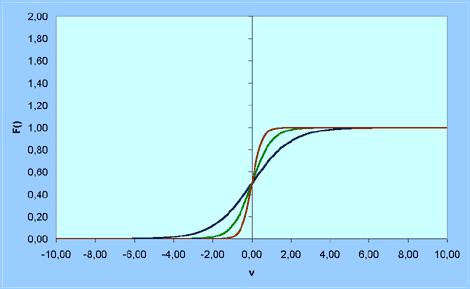F(v) = 1 / (1 + exp(-av)), where a is the slope parameter.
Three examples with different a are given on a chart.

The sigmoid function above is known as "logistic function", but there are other forms of sigmoid function, too. For example:
F(v) = tanh(v / 2) = (1 - exp(-v)) / (1 + exp(-v))

Sigmoid functions are the most common activation functions used in artificial neural networks.

Artificial neural network

As already mentioned before, there is a plethora of different network models, and the level of similarity is considerably lower than it was in artificial neurons. We can compare the neuron with a building block -- by using standard blocks it is possible to build a great number of houses in different styles. Start customizing your blocks and the possibilities jump to infinity.

The most common class of ANNs is multilayer feedforward networks: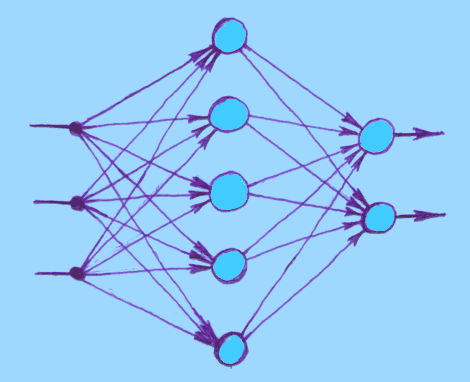It has a layered structure. Input nodes, whose only purpose is to distribute the input values into network, form an input layer (on the left on picture). All other nodes are artificial neurons, which also form layers: the output layer (on the right), and between input and output layers is one or more "hidden" layers. "Feedforward" implies that signals move only towards outputs -- no feedback loops are present. Network on the picture is "fully connected" in the sense that every neuron in each layer is connected to every other node in its neighbouring layers. If some connections were missing, it would be a "partially connected" network.

Training a network

If you have carefully followed the story so far, you probably already have some ideas about how ANN will work: it is given a bunch of input values, it processes them and gives back some output values. Clean and simple :) Except one problem -- we would like the ANN to have a REASONABLE reaction to given inputs, not just any random reaction! That's were training comes in.

As there are different architectures of networks, there are also appropriate training algorithms for each of them. From network's point of view it would make more sense to call them learning algorithms, especially because some of them do not require any teacher (unsupervised learning). However, in case of multilayer feedforward networks a supervised learning procedure is used most often, usually a "backpropagation" algorithm. Its principle is:

• Input values are fed into network.
• Network's reaction (output values) is read out and compared to the desired reaction.
• Error values are calculated out of the difference between desired and actual outputs using some error function.
• The weights of connections owned by output layer are adjusted according to the error values.
• Error values are then BACKpropagated to the hidden layer next to output layer, and its weights are adjusted.
• Backpropagation and weight adjustment is repeated until input layer is reached.
• The whole procedure is repeated until error is acceptably small.

The point of using ANNs is that although we train them only with a few training patterns (which consist of input values and desired output values for these inputs), the ANN can also correspond reasonably to the inputs that are similar to the learned ones, but not exactly the same. This is called "function approximation" and is quite useful in many applications.

The details of training procedure usually contain heavy math and are not easily grasped. Following is just a short overview about HOW the backpropagation can be done. Answering the question "WHY it can be done that way" would definitely be more reasonable and enlightening, but I'm currently not up to the task of doing it, especially in a user friendly way :) Anyway, if you get an intense feeling of repulsion when looking at the following section, then feel free to skip it and scroll down to the next paragraph.

First of all, the calculation of output values is done using the neuron model descibed above (input values are set into the input layer; then all neurons in first hidden layer are updated according to the neuron model; then neurons in second hidden layer; ... ; until output layer is reached).

Now we have output values for this given set of input values, but most likely they are not what we want them to be. So we can calculate error value for each neuron in the output layer.

error_value = desired_output - actual_output

Then an error signal is calculated for each of these neurons:
error_signal = error_value * F ' (v)

where v is the adjusted sum in neuron (see the neuron model above) and F ' (v) is the derivative of activation function F() in point v.

And then all weights owned by these neurons are updated:
w1 = w1 + learning_rate * error_signal * x1
w2 = w2 + learning_rate * error_signal * x2
...
where x1, x2, ... are inputs into this neuron from neurons located in previous layer (once again, see the neuron model above) and learning_rate is a parameter affecting the speed of learning (it should be noted that it is also a "forgetting speed" in some sense -- if learning_rate is very high, then network quickly learns new training patterns, but on the cost of forgetting others; plus additional danger that ANN becomes so instable it can't learn any single pattern at all, like a hyperactive child.).

Now the weights of output layer are updated, but what about other layers? We can't repeat exactly the same procedure because we don't have desired output values for hidden layers. The solution is BACKpropagation of the error from output layer into hidden layers.To get the error_value for a neuron that is NOT in output layer, the following formula can be used:
error_value = wo1* es1 + wo2*es2 + ... + won*esn

where "wo" is the weight of a connection between current neuron and a neuron in following layer (see the picture above), and "es" is the error_signal of that other neuron.

Formulas for calculating the error_signal and new weights are here exactly the same as they were for output neurons.

This backpropagation of error and weights updating is done all the way through network till input layer is reached. Then the whole process is repeated until error is small enough. If there is more than one training pattern, then they are used by turns: inputs of the first pattern are fed into network, error is calculated, weights are adjusted; inputs of the second pattern are fed into network, error is calculated, weights are adjusted; ... ; then again the first pattern, second pattern, ... .

It is quite likely that you have at least one question after reading previous algorithm: what exactly is the derivative of F(v) and why is it used anyway? It should be clear that F ' (v) is not the same for all activation functions F(v). One of the most common activation functions is F(v) = 1 / (1 + exp(-av)) and it's derivative is mostly given in a form F ' (v) = a * y * (1 - y), where y = F(v) (y is the output of the neuron, see neuron model above). It may be somewhat surprising first, but if you take a few minutes and try it on a paper then it makes more sense. First you get a big messy derivative, but some replacements should reduce it down to given short form which is much easier to use than the big messy one. Why is derivative needed, anyway? Because backpropagation is a gradient descent algorithm, meaning it tries to move the system towards the deepest descent on error surface for getting the fastest reduction of error. But finding the deepest descent of a surface is, naturally, done by using gradient, which in turn is found by using derivatives. As simple as that :) Feel free to search for additional information from Internet or books.

What can be done with ANNs?

As we have now seen, artificial neural networks are very far from the complexity of their biological counterparts. There is also an additional problem -- in brain all neurons are working all the time (in parallel), but our computers work in sequential fashion, meaning they have to update artificial neurons one by one which is an awfully time-consuming process. Special hardware can be built for ANNs, but often it would be too expensive. So a question rises: do we need ANNs at all? Where?

The answer is: although ANNs probably are NOT the hoped breakthrough in Artificial Life research, they do have turned out to be very useful in many fields of technology. ANNs have been successfully used in various signal processing applications from sonar and radar classificators to word recognizers. They have a lot of use in industry: process control, quality prediction and inspection systems, process and machine diagnosis, etc. In robotics ANNs are used for vision systems and for controlling the robot's movements. Business applications include price prediction programs for stocks and currencies. ANNs can also be used in computer games and animations for various purposes like controlling the character movement or doing some other necessary decisions.

Summary

This was just a short introduction to the field of ANNs. Only one neuron model, one network architecture and one training algorithm were described and even this was done without much details. Currently I do NOT have any plans to write more essays on standard ANNs, so this page is unlikely to be filled with any further information. However, there is a great variety of materials available on Internet and in books, for beginners and for professionals. If you DO have interest in the topic, please take some time and look for more information. A few links are provided in Links section, accessible from the front page. But before clicking away towards the shining mountains of wisdom, please check out some educational software based on the same models described in this essay: see the Software section on the front page.
Back | Home